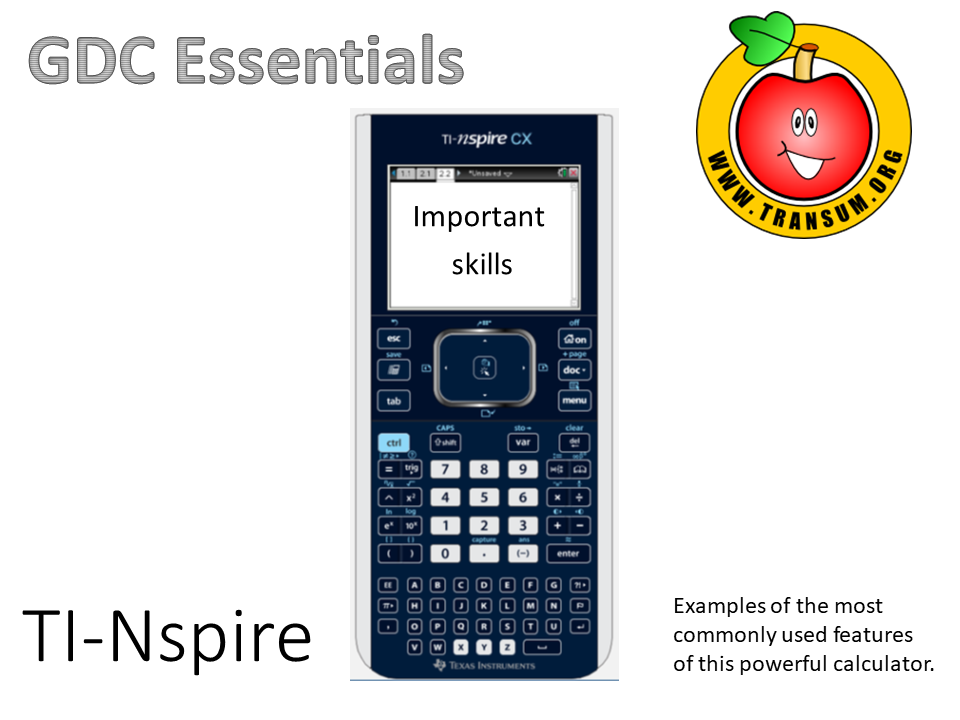Here is the URL for some more practice using your calculator.

Transum.org/go/?to=UsingCalc

TI-Nspire CX Essential Skills (45 slides)

Learn a skill a day for twenty days. There is a printable checklist to go with this presentation.Go directly to a particular skill:

Day 1 Solve simultaneous equations
Day 2 Solve quadratic equations
Day 3 Standard form and variables
Day 4 Defining a function
Day 5 Generating a sequence
Day 6 Calculating statistics from a list
Day 7 Calculating statistics from a frequency table
Day 8 Box plot from frequency table
Day 9 Plotting a graph and rescaling axes
Day 10 Finding a zero of a line
Day 11 Solving simultaneous equations graphically
Day 12 Finding local maximum and minimum points
Day 13 Exponential graphs and asymptotes
Day 14 Using nSolve to find a specific solution
Day 15 Scatter graphs and regression lines
Day 16 Spearman's rank correlation coefficient
Day 17 Using the normal distribution
Day 18 Using the binomial distribution
Day 19 The chi squared test for independence
Day 20 Using the finance solver

Go directly to a particular slide:

This presentation as a PowerPoint file is available to teachers who have subscribed to Transum.

Here is the URL of this presentation linking directly to the slide you are currently viewing, slide number 1. Copy and paste this link into your scheme of work, revision notes or blog.

There are many more mathematical visual aids to project onto a whiteboard or screen in our Shine+Write area.